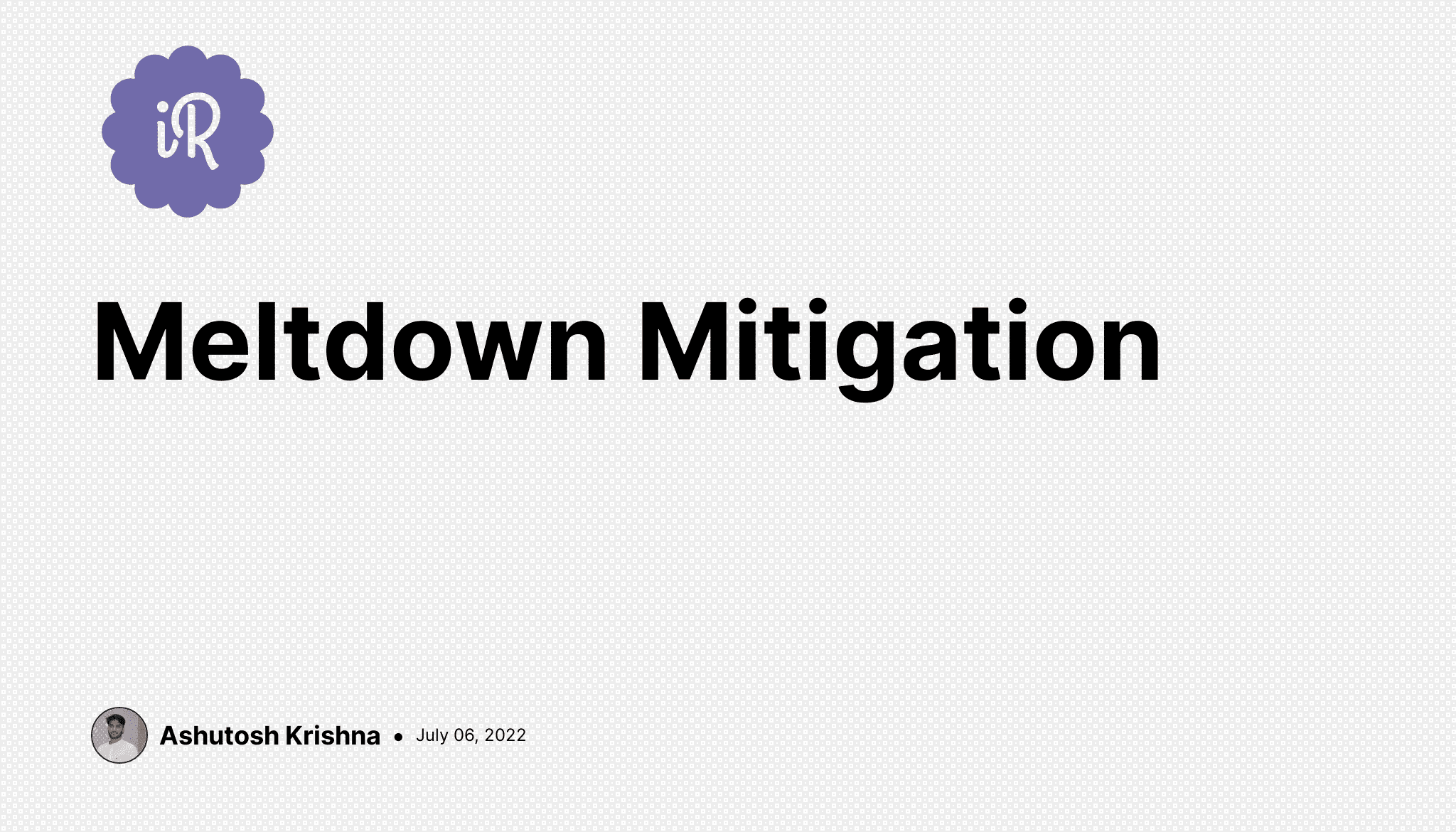# Ashutosh Writes# Meltdown Mitigation

Instructions

In this exercise, we'll develop a simple control system for a nuclear reactor.

For a reactor to produce the power it must be in a state of criticality. If the reactor is in a state less than criticality, it can become damaged. If the reactor state goes beyond criticality, it can overload and result in a meltdown. We want to mitigate the chances of meltdown and correctly manage reactor state.

The following three tasks are all related to writing code for maintaining ideal reactor state.

#### 1. Check for criticality

The first thing a control system has to do is check if the reactor is balanced in criticality. A reactor is said to be critical if it satisfies the following conditions:

• The temperature is less than 800 K.
• The number of neutrons emitted per second is greater than 500.
• The product of temperature and neutrons emitted per second is less than 500000.

Implement the function `is_criticality_balanced()` that takes `temperature` measured in kelvin and `neutrons_emitted` as parameters, and returns `True` if the criticality conditions are met, `False` if not.

``````>>> is_criticality_balanced(750, 600)
True
``````

#### 2. Determine the Power output range

Once the reactor has started producing power its efficiency needs to be determined. Efficiency can be grouped into 4 bands:

1. `green` -> efficiency of 80% or more,
2. `orange` -> efficiency of less than 80% but at least 60%,
3. `red` -> efficiency below 60%, but still 30% or more,
4. `black` -> less than 30% efficient.

The percentage value can be calculated as `(generated_power/theoretical_max_power)*100` where `generated_power` = `voltage` * `current`. Note that the percentage value is usually not an integer number, so make sure to consider the proper use of the `<` and `<=` comparisons.

Implement the function `reactor_efficiency(<voltage>, <current>, <theoretical_max_power>)`, with three parameters: `voltage`, `current`, and `theoretical_max_power`. This function should return the efficiency band of the reactor : 'green', 'orange', 'red', or 'black'.

``````>>> reactor_efficiency(200,50,15000)
'orange'
``````

#### 3. Fail Safe Mechanism

Your final task involves creating a fail-safe mechanism to avoid overload and meltdown. This mechanism will determine if the reactor is below, at, or above the ideal criticality threshold. Criticality can then be increased, decreased, or stopped by inserting (or removing) control rods into the reactor.

Implement the function called `fail_safe()`, which takes 3 parameters: `temperature` measured in kelvin, `neutrons_produced_per_second`, and `threshold`, and outputs a status code for the reactor.

• If `temperature * neutrons_produced_per_second` < 90% of `threshold`, output a status code of 'LOW' indicating that control rods must be removed to produce power.
• If `temperature * neutrons_produced_per_second` are within plus or minus 10% of the `threshold` the reactor is in criticality and the status code of 'NORMAL' should be output, indicating that the reactor is in optimum condition and control rods are in an ideal position.
• If `temperature * neutrons_produced_per_second` is not in the above-stated ranges, the reactor is going into meltdown and a status code of 'DANGER' must be passed to immediately shut down the reactor.
``````>>> fail_safe(temperature=1000, neutrons_produced_per_second=30, threshold=5000)
'DANGER'
``````

## Solution

``````"""Functions to prevent a nuclear meltdown."""

def is_criticality_balanced(temperature, neutrons_emitted):
"""Verify criticality is balanced.

:param temperature: int or float - temperature value in kelvin.
:param neutrons_emitted: int or float - number of neutrons emitted per second.
:return: bool - is criticality balanced?

A reactor is said to be critical if it satisfies the following conditions:
- The temperature is less than 800 K.
- The number of neutrons emitted per second is greater than 500.
- The product of temperature and neutrons emitted per second is less than 500000.
"""

return temperature < 800 and neutrons_emitted > 500 and temperature * neutrons_emitted < 500000

def reactor_efficiency(voltage, current, theoretical_max_power):
"""Assess reactor efficiency zone.

:param voltage: int or float - voltage value.
:param current: int or float - current value.
:param theoretical_max_power: int or float - power that corresponds to a 100% efficiency.
:return: str - one of ('green', 'orange', 'red', or 'black').

Efficiency can be grouped into 4 bands:

1. green -> efficiency of 80% or more,
2. orange -> efficiency of less than 80% but at least 60%,
3. red -> efficiency below 60%, but still 30% or more,
4. black ->  less than 30% efficient.

The percentage value is calculated as
(generated power/ theoretical max power)*100
where generated power = voltage * current
"""
generated_power = voltage * current
efficiency = (generated_power/theoretical_max_power) * 100
if efficiency >= 80:
return 'green'
if efficiency >= 60:
return 'orange'
if efficiency >= 30:
return 'red'
return 'black'

def fail_safe(temperature, neutrons_produced_per_second, threshold):
"""Assess and return status code for the reactor.

:param temperature: int or float - value of the temperature in kelvin.
:param neutrons_produced_per_second: int or float - neutron flux.
:param threshold: int or float - threshold for category.
:return: str - one of ('LOW', 'NORMAL', 'DANGER').

1. 'LOW' -> `temperature * neutrons per second` < 90% of `threshold`
2. 'NORMAL' -> `temperature * neutrons per second` +/- 10% of `threshold`
3. 'DANGER' -> `temperature * neutrons per second` is not in the above-stated ranges
"""

value = temperature * neutrons_produced_per_second
if value < threshold * 0.9:
return 'LOW'
if value > threshold * 1.1:
return 'DANGER'
return 'NORMAL'
``````